# Skolem function

A concept in predicate logic. If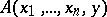is a predicate formula with individual variables (cf. Individual variable), whose domains are sets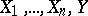, respectively, then a function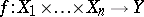is called a Skolem function or resolving function for the formula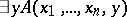if for all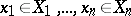one has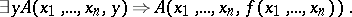Skolem functions were introduced by T. Skolem in the 1920s and have since been widely used in papers on mathematical logic. This is due to the fact that Skolem functions can be used to eliminate alternation of the quantifiers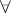and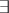. For example, for every formulaof the language of the restricted predicate calculus it is possible to construct a formula of the form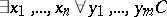(called the Skolem normal form of), wheredoes not contain new quantifiers but does contain new function symbols, such thatis deducible in predicate calculus if and only if its Skolem normal form is.
By way of an example one can point to Jensen's result on the deducibility of Chang's two-cardinals conjecture (see ) and the negation of the Suslin hypothesis (see ) from Gödel's axiom of constructibility. The Novikov–Kondo theorem on the uniformization of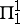-relations from descriptive set theory confirms the existence of a certain type of Skolem function (see ).